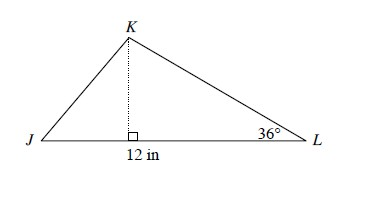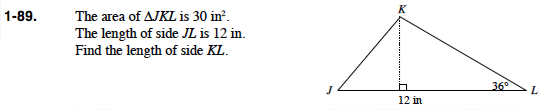### Home > PC > Chapter 1 > Lesson 1.2.2 > Problem1-89

1-89.

The area of ΔJKL is 30 in2. The length of side JL is 12 in. Find the length of side KL. Homework Help ✎Label the height.

Since the area of the triangle is given. What is the area of any triangle?

$\frac{1}{2}\text{(base)(height)}$

Find h. Then find length KL by using the sine function.

$\approx 8.51\text{ inches}$